# Ordering Scheme

Let us assume that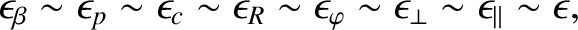(8.47)

where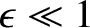.

Table 5.1 gives estimates for the values of the various parameters that characterize the rescaled reduced drift-MHD model, (8.16)–(8.20), in a low-field and a high-field tokamak fusion reactor. (See Chapter 1.) These estimates are made using the following assumptions: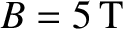(low-field) or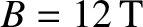(high-field),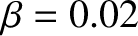,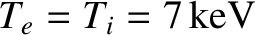,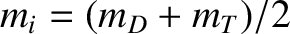(where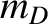and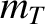are the deuteron and triton masses, respectively),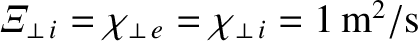,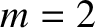,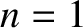,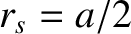(where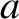is the minor radius of the plasma),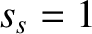,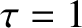,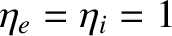, and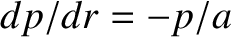. It is clear from Table 5.1 and Equations (8.25)–(8.34) that all of the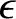parameters appearing in the rescaled, reduced, drift-MHD equations are less than unity, in accordance with Equation (8.47), provided that the radial width of the magnetic island chain that develops in the inner region exceeds a few centimeters.

Table: 8.1 Parameters characterizing the rescaled reduced drift-MHD model for a low-field and a high-field tokamak reactor. [See Equations (8.23)–(8.34) and (8.84).]
 Low-Field High-Field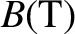5.0 12.0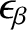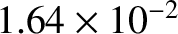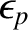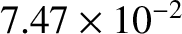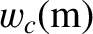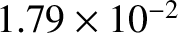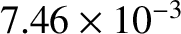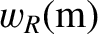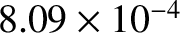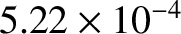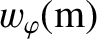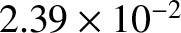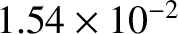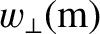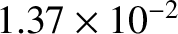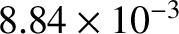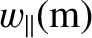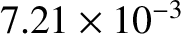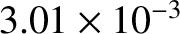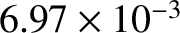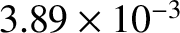The orderings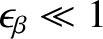and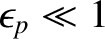are a direct consequence of the fact that conventional tokamak fusion reactors have large aspect-ratios (i.e.,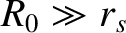), and confine low-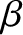plasmas (i.e.,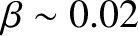). As will become apparent, the ordering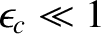ensures that the island chain is sufficiently wide that ion acoustic waves propagating parallel to the magnetic field are able to smooth out any variations in the normalized plasma pressure,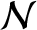, around magnetic flux-surfaces . The orderings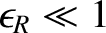,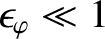, and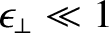ensure that the island chain is sufficiently wide that the perpendicular diffusion of magnetic flux, momentum, and energy are not dominant effects in the rescaled, reduced, drift-MHD equations. Finally, the ordering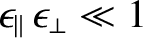ensures that the island chain is sufficiently wide that parallel energy transport smooths out any variations in the normalized plasma pressure,, around magnetic flux-surfaces .

Finally, suppose that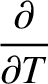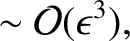(8.48)

and let us expand the various fields in our rescaled model as follows: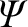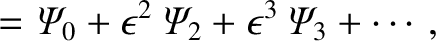(8.49)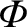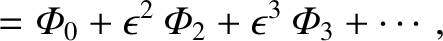(8.50)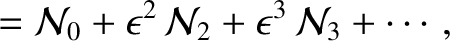(8.51)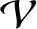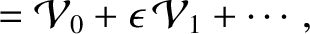(8.52)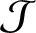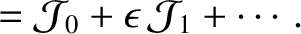(8.53)

Here,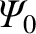,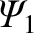, et cetera are assumed to be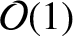in the inner region.